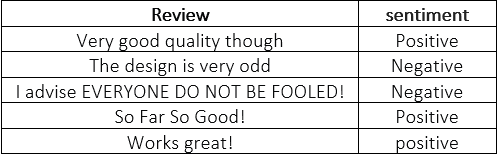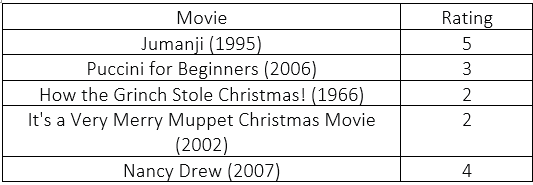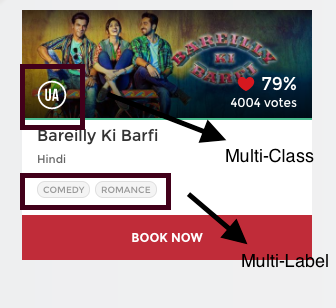CHIRAG GOYAL — Published On July 20, 2021 and Last Modified On August 26th, 2021

This article was published as a part of the Data Science Blogathon

## Introduction

While solving the classification problem statements using Deep Learning, we may come up with mainly the following two types of classification tasks:

• Multi-Class Classification
• Multi-Label Classification

As a short introduction, In multi-class classification, each input will have only one output class, but in multi-label classification, each input can have multi-output classes.

Image Source:

But these terms i.e, Multi-class and Multi-label classification can confuse even the intermediate developer. So, In this article, I have tried to give you a clear and easy intuition with examples of these terms in a detailed manner. If you are a Data Science Enthusiast, then read this article completely and accelerate your Data Science Journey.

• What is Binary Classification?
• What is Multi-Class Classification?
• What is Multi-Label Classification?
• A Real-Life example to understand the difference between multi-class and multi-label classification
• Test Your Knowledge (Interview Quiz)

## What is Binary Classification?

In binary classification problem statements, any of the samples from the dataset takes only one label out of two classes.

For example, Let’s see an example of small data taken from amazon reviews data set.Table Showing an Example of Binary Classification Problem Statement

Image Source:

If we carefully look into the table, we will see that we can only classify the review as either positive or negative i.e, only two possible target outcomes. So, this is an example of a binary classification problem statement.

## What is Multi-Class Classification?

To understand multi-class classification, firstly we will understand what is meant by multi-class, and find the difference between multi-class and binary-class.

Multi-class vs. binary-class is the issue of the number of classes your classifier will be modeling. Theoretically, a binary classifier is much less complicated than a multi-class classifier, so it is essential to make this distinction.

For example, the Support Vector Machine (SVM) trivially can learn one hyperplane to split two classes, but 3 or more classes make it complex. In neural networks, we usually use the Sigmoid Activation Function for binary classification tasks while on the other hand, we use the Softmax activation function for multi-class as the last layer of the model.

For multi-class classification, we need the output of the deep learning model to always give exactly one class as the output class.

For example, If we are making an animal classifier that classifies between Dog, Rabbit, Cat, and Tiger, it makes sense only for one of these classes to be selected each time.

Image Source:

To ensure only one class is selected each time, we apply the Softmax Activation Function at the last layer and we use log loss to train the model.

Therefore, for a given dataset, any of the samples that come from the dataset takes only one label out of the number of classes. Let’s see an example of small data taken from the movies reviews dataset.Table Showing an Example of Multi-Class Classification Problem Statement

Image Source:

If we carefully look into the table, we will see that we can only classify the movie rating from 2 to 5 i.e, each movie will have only one label (2, 3, 4, or 5). This means samples can have more than two possible target outcomes. So, this is an example of a multi-class classification problem statement.

## What is Multi-Label Classification?

To understand multi-label classification, firstly we will understand what is meant by multi-label, and find the difference between multi-label and binary-label.

Multi-label vs. single-label is the matter of how many classes an object or example can belong to. In neural networks, when single-label is required, we use a single softmax layer as the last layer, learning a single probability distribution that ranges over all classes. In the case where multi-label classification is needed, we use multiple sigmoids on the last layer and thus learn a separate distribution for each class.

In certain problems, each input can have multiple, or even none, of the designated output classes. In these cases, we go for the multi-label classification problem approach.

For example, If we are building a model which predicts all the clothing articles a person is wearing, we can use a multi-label classification model since there can be more than one possible option at once.

Image Source:

Therefore, for a given dataset, any of the samples that come from the dataset takes more than one label out of the number of available classes. Let’s see a toy example.Table Showing an Example of Multi-Label Classification Problem Statement

Image Source:

If we carefully look into the table, we will see that the movie may take more than one genre i.e, the movie could be comedy and Fantasy at the same time. This means samples can have more than two possible labels. So, this is an example of a multi-label classification problem statement.

## Real-Life Example to Understand the Difference between Multi-Class and Multi-Label Classification

Consider the following real-life example to understand the difference between these two types of classification. To understand the exact difference, I hope the below image makes things quite clear. Let’s try to understand it.Image Source:

As you can know the general information that for any movie, the organization named Central Board of Film Certification, issues a certificate depending on the contents of the movie.

For example, if you look in the above image, then you may see that this movie has been rated as ‘U/A’ (meaning ‘Parental Guidance for children below the age of 12 years) certificate. This is not the only type of certificate but there are other types of certificates classes such as,

• ‘A’ (Restricted to adults), or
• ‘U’ (Unrestricted Public Exhibition),

but while categorizing the movies based on this, it is sure that each movie can only be categorized with only one out of those three types of certificates. In short, there are multiple categories (i.e, multiple certificates assigned to the movie) but each instance is assigned only one (i.e, each movie is assigned with only one certificate at once), therefore such problems are categorized under the multi-class classification problem statement.

Again, if you see carefully the image, then this movie has been categorized into the comedy and romance genres. But this time there is a difference that each of the movies can fall into one or more different sets of categories (i.e, have more than one genre). Therefore, each instance can be assigned with multiple categories (i.,e multiple genres), so these types of problems are categorized under the multi-label classification problem statement, where we have a set of target labels for each of the samples.

Great! after understanding this example properly, now you can easily distinguish between multi-label and multi-class problem statements. Congratulations on this! 😊

## Test Your Knowledge (Interview Quiz)

In this section, I have given some questions to test your knowledge regarding the topic which we have discussed in this article.

Question-1: Multi-class classification problems have multiple categories but each instance is assigned only once.

• True
• False

Question-2: Multi-label classification problems have each instance can be assigned with multiple categories or a set of target labels.

• True
• False

NOTE: Feel free to discuss the answer to these questions in the comment box below!

## Self Learning Resource

If you want to know how to solve multiclass and multilabel classification problem statements, you can refer to the following link.

## Other Blog Posts by Me

You can also check my previous blog posts.

Previous Data Science Blog posts.

Here is my Linkedin profile in case you want to connect with me. I’ll be happy to be connected with you.

## Email

For any queries, you can mail me on Gmail.

## End Notes